## Example Questions

2 Next →

### Example Question #11 : How To Find A Reference Angle

What is the reference angle for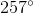?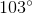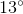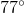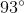Explanation:

A reference angle is the smallest possible angle between a given angle measurement and the x-axis.

In this case, our anglelies in Quadrant III, so the angle is found by the formula.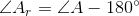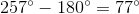Thus, the reference angle foris.

### Example Question #12 : How To Find A Reference Angle

What is the reference angle for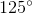?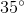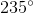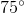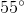Explanation:

A reference angle is the smallest possible angle between a given angle measurement and the x-axis.

In this case, our anglelies in Quadrant II, so we can find our reference angle using the formula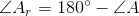.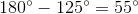Thus, the reference angle foris.

2 Next →